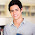## Monday, July 9, 2012

### The GeoGebra Interface

The GeoGebra window as shown below is capable of simultaneously displaying algebraic, geometric, and numeric representations of mathematical objects. A function, for example, can be viewed as a graph, an equation, and a table.Parts of GeoGebra
• Menu bar - used for managing files, modifying preferences, and customizing settings.
• Toolbar - used for constructing and modifying mathematical objects.
• Graphics view - used for displaying geometric representations of mathematical objects
• Algebra view - used for displaying algebraic representations of mathematical objects.
• Input bar - used for inputting equations and performing mathematical computations

1.2.3.